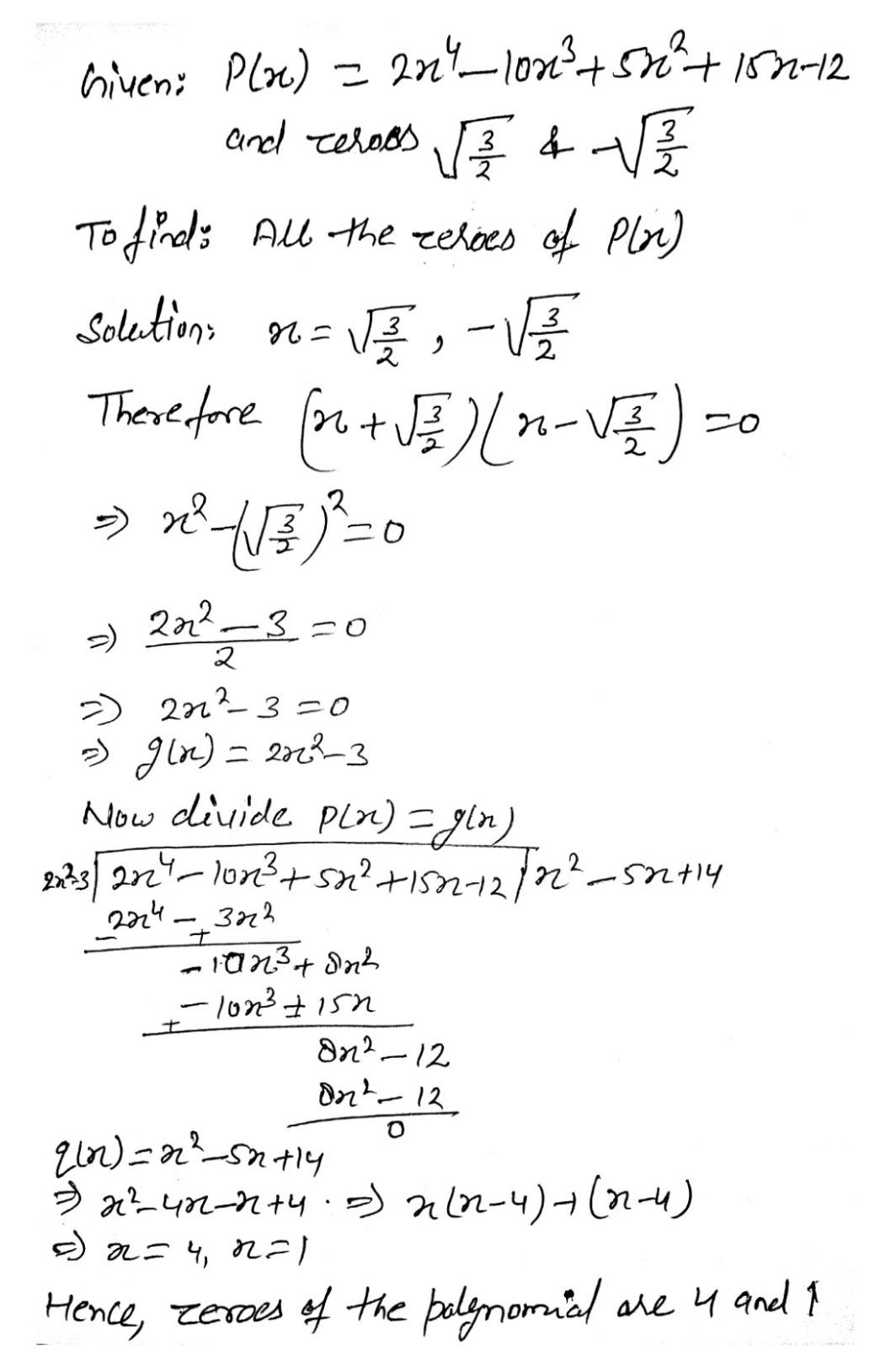Symbol
ProblemFind all the zeroes of the polynomials $2x^{4}-10x^{3}+5x^{2}+15x-12$ if it is given that one of it $2eFQ$ is $\sqrt{\dfrac {3} {2}}$ $3$ $\left(~^{2}$ $4°$ $\left(2$